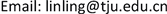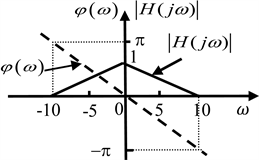﻿ “电路、信号与系统”中的相量和频域分析教学方法研究 Phasor Analysis and Frequency Domain Analysis in Circuits, Signals and Systems

Creative Education Studies
Vol. 07  No. 05 ( 2019 ), Article ID: 32782 , 7 pages
10.12677/CES.2019.75115

Phasor Analysis and Frequency Domain Analysis in Circuits, Signals and Systems

Ling Lin*, Zhoumo Zeng, Dachao Li, Yu Zhang, Rong Liu, Chunmei Yang

School of Precision Instrument and Opto-Electronics Engineering, Tianjin University, TianjinReceived: Oct. 8th, 2019; accepted: Oct. 24th, 2019; published: Oct. 31st, 2019ABSTRACT

In the courses of “Circuit Basic Theory” and “Signal and System”, the sinusoidal steady-state analysisof circuit based on phasor methodand the frequency domain analysis of system both are important contents, and there is a deep internal relation between them, which few students can really understand. Based on the analysis of the essence, similarities and differences of the phasor method and the frequencydomain method，as well as the problems encountered during teaching activities, this paper puts forward a new teaching idea, hoping to improving the teaching effectiveness for the course of “Circuits, Signals and Systems”.

Keywords:Circuit, Signal and System, Phasor Method, Frequency Domain Analysis

“电路、信号与系统”中的相量和频域分析 教学方法研究1. 引言

2. 传统电路理论课程中相量法

3. 信号与系统课程中的频域分析方法

“信号与系统”对信号和系统给出了更一般化的描述，信号的时域、频域与S域分解，系统的时域、频域与S域分析，依次展开，层层推进，概念丰富、逻辑清晰、理论完整，无疑在更高的层面上提供了分析包括电路系统在内的动态系统的理论方法。然而，多年的教学实践中注意到，学生对于把握信号系统框架中时域、频域与S域三类不同分析方法的本质与内在关系感到困难，比较容易停留在纯粹的数学变换层面；并且对“信号与系统”和“电路基础理论”中相关联的内容，如系统稳态响应分析以及系统频响特性，与“电路基础理论”中正弦稳态分析部分的关系认识不到位，导致花费了大量的课堂资源，却没有收到应有的教学效果。例如，已知系统频率响应函数 $H\left(j\omega \right)$图1所示，当 $f\left(t\right)=2+4\mathrm{cos}\left(5t\right)+4\mathrm{cos}\left(10t\right)$ 时，求系统响应。Figure 1. Amplitude and phase frequency characteristics of a system

$f\left(t\right)=2+4\mathrm{cos}\left(5t\right)+4\mathrm{cos}\left(10t\right)=\underset{n=-2}{\overset{2}{\sum }}2{\text{e}}^{jn5t}$,

$\begin{array}{c}y\left(t\right)=\underset{n=-2}{\overset{2}{\sum }}{Y}_{n}{\text{e}}^{jn5t}\\ =0+{\text{e}}^{-j\left(5t-{90}^{\circ }\right)}+2+{\text{e}}^{j\left(5t-{90}^{\circ }\right)}+0\\ =2+2\mathrm{cos}\left(5t-{90}^{\circ }\right)\end{array}$

$Y\left(j\omega \right)=4\pi \left[0.5{\text{e}}^{j{90}^{\circ }}\delta \left(\omega +5\right)+\delta \left(\omega \right)+0.5{\text{e}}^{-j{90}^{\circ }}\delta \left(\omega -5\right)\right]$,

$y\left(t\right)={\text{e}}^{-j\left(5t-{90}^{\circ }\right)}+2+{\text{e}}^{j\left(5t-{90}^{\circ }\right)}=2+2\mathrm{cos}\left(5t-{90}^{\circ }\right)$.

“信号与系统”作为一门独立的课程是在“电路基础理论”之后 ，其中系统频域分析部分与“电路基础理论”中的相量法以及相关的阻抗、网络函数等概念之间的有着深刻的内在联系。然而教学中发Figure 2. Periodic square wave signal acts on the RL circuit

1) 求出 ${u}_{s}\left(t\right)$ 的前五次谐波表达式：

${{u}_{s}\left(t\right)|}_{0,\sim ,5}=\frac{1}{2}+\frac{2}{\pi }\mathrm{cos}\left(t\right)-\frac{2}{3\pi }\mathrm{cos}\left(3t\right)+\frac{2}{5\pi }\mathrm{cos}\left(5t\right)$ ;

2) 列写微分方程 ${i}^{\prime }\left(t\right)+i\left(t\right)={u}_{s}\left(t\right)$

3) 设 $i\left(t\right)$ 的前五次谐波为

${C}_{0}+{C}_{1}\mathrm{cos}\left(t\right)+{C}_{2}\mathrm{sin}\left(t\right)+{C}_{3}\mathrm{cos}\left(3t\right)+{C}_{4}\mathrm{sin}\left(3t\right)+{C}_{5}\mathrm{cos}\left(5t\right)+{C}_{6}\mathrm{sin}\left(5t\right)$

4) 代入微分方程求得7个待定系数;

5) 最后得到 $i\left(t\right)$ 的前五次谐波为

$\frac{1}{2}+\frac{1}{\pi }\mathrm{cos}\left(t\right)+\frac{1}{\pi }\mathrm{sin}\left(t\right)-\frac{1}{15\pi }\mathrm{cos}\left(3t\right)-\frac{1}{5\pi }\mathrm{sin}\left(3t\right)+\frac{1}{65\pi }\mathrm{cos}\left(5t\right)+\frac{1}{13\pi }\mathrm{sin}\left(5t\right)$

4. 电路、信号与系统课程中的系统稳态分析

“电路基础理论”与“信号与系统”两门课的结合点在于动态电路的分析方法。笔者之前提出了“电路、信号与系统”课程的总体组织框架 ，即，电路篇、信号篇和系统篇。基本思想是，在电路篇里在电阻电路的环境下介绍电路所特有的基本规律、定理和分析方法；在动态电路部分先介绍基本的动态元件(电感、电容等)的伏安关系，动态电路微分方程的列写以及响应的构成，强调动态电路所具有的动态响应和稳态响应过程。电路正弦稳态分析则从电路篇中移出，合并到系统篇中的系统稳态分析部分，在频域分析方法之后引出。在信号篇里，以线性叠加定理为切入点，系统介绍信号的时域、频域以及s域的分解。在强调积分、傅氏和拉氏三大变换的物理意义的同时为解决动态系统分析提供必要的数学工具。在系统篇里则打破时域、频域以及s域分析的传统框架，将系统篇分为系统动态分析和稳态分析两个部分。灵活应用数学工具为系统分析服务。比如在系统动态分析部分，直接把时域和S域方法打通。引入电路的S域模型，强调指出，在S域模型下，之前电路篇中的电阻电路的理论、分析方法可以完全移植过来，从而方便地解决动态电路的动态分析。在动态过程分析中强调系统过渡过程和稳态响应的概念，为系统稳态分析做好铺垫。

$\begin{array}{c}{y}_{1}\left(t\right)={f}_{1}\left(t\right)\ast h\left(t\right)={\int }_{-\infty }^{+\infty }A{\text{e}}^{j\left[{\omega }_{0}\left(t-\tau \right)+{\phi }_{0}\right]}h\left(\tau \right)\text{d}\tau \\ =A{\text{e}}^{j\left({\omega }_{0}t+{\phi }_{0}\right)}{\int }_{-\infty }^{+\infty }A{\text{e}}^{-j{\omega }_{0}\tau }h\left(\tau \right)\text{d}\tau \\ =A{\text{e}}^{j\left({\omega }_{0}t+{\phi }_{0}\right)}H\left(j{\omega }_{0}\right)\\ =A|H\left(j{\omega }_{0}\right)|{\text{e}}^{j\left({\omega }_{0}t+{\phi }_{0}\right)}{\text{e}}^{j\phi \left({\omega }_{0}\right)}\\ =A|H\left(j{\omega }_{0}\right)|{\text{e}}^{j\left[{\omega }_{0}t+{\phi }_{0}+\phi \left({\omega }_{0}\right)\right]}\end{array}$

${Y}_{1}\left(s\right)=\frac{s}{{s}^{2}+{\omega }_{0}^{2}}H\left(s\right)={Y}_{1h}\left(s\right)+\frac{1}{2}\frac{1}{s-j{\omega }_{0}}H\left(j{\omega }_{0}\right)+\frac{1}{2}\frac{1}{s+j{\omega }_{0}}H\left(-j{\omega }_{0}\right)$

5. 总结

“电路基础理论”和“信号与系统”两门课程连接点在于动态电路的分析。在多数“电路基础理论”教材中，是以分析对象、分析任务为“导向”，在需要的地方引入相应的数学知识，使学习者往往因为零散出现的数学问题而感到困惑；而“信号与系统”更偏重于理论方法的系统完整性，使得一些学习者不自觉地陷入数学推演里，从而忽视了理论的工程背景及其实际应用。因此，当把“电路基础理论”和“信号与系统”两门课程整合到一起时，应该在一个更高的层面审视两门课程的理论体系，寻求更加合理、更加高效的课程组织体系和教学方法，让学生在更少的课堂学时资源的情况下，更好地掌握电路与信号系统的核心理论并能够灵活地应用于解决实际问题。本文提出的关于频域分析和相量法的教学方法便是研究两门课程内在关系的一个实例。

Phasor Analysis and Frequency Domain Analysis in Circuits, Signals and Systems[J]. 创新教育研究, 2019, 07(05): 679-685. https://doi.org/10.12677/CES.2019.75115

1. 1. (美)亚历山大. 电路基础(英文版)[M]. 第五版. 北京: 机械工业出版社, 2013.

2. 2. 郑君里, 谷源涛. 信号与系统课程历史变革与进展[J]. 电气电子教学学报, 2012, 34(2): 1-6.

3. 3. 林凌, 曾周末, 栗大超, 张宇, 刘蓉. “电路、信号与系统”课程内容组织体系[J]. 电气电子教学学报, 2018, 40(5): 52-56.

NOTES

*第一作者。